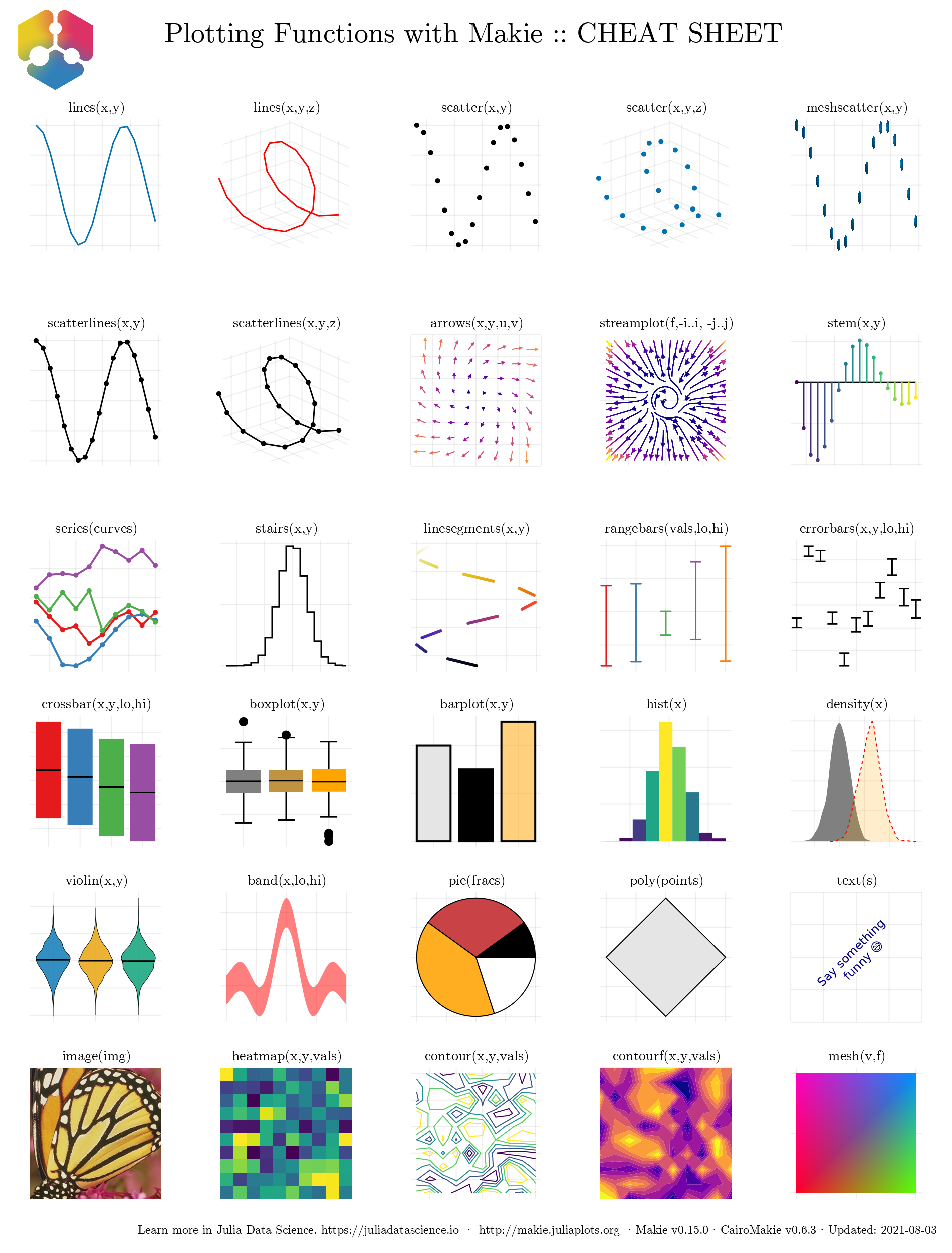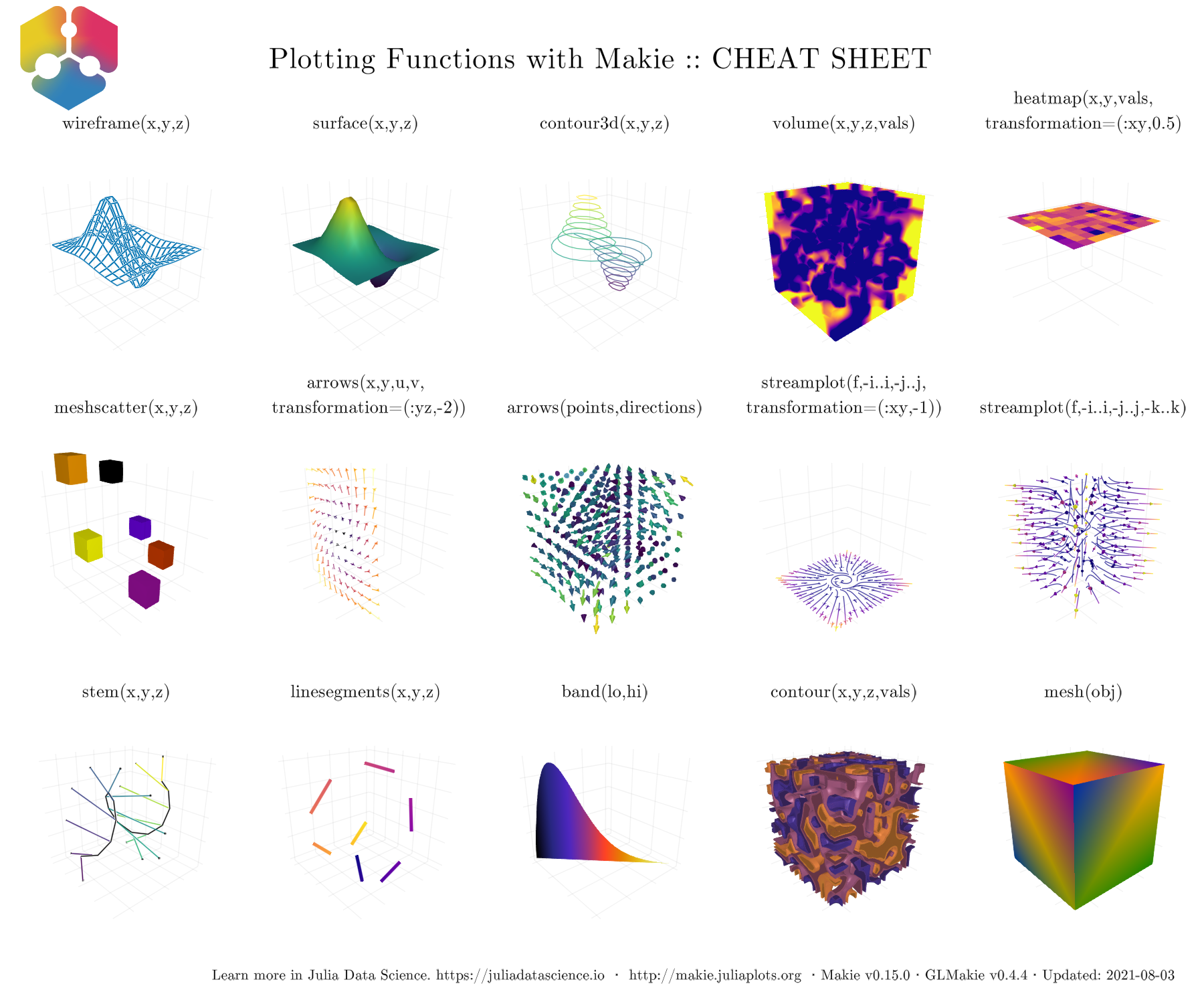# Makie

A data visualization ecosystem for Julia

Author

Marie-Hélène Burle

There are several popular data visualization libraries for the Julia programming language (e.g. Plots, Gadfly, VegaLite, Makie). They vary in their precompilation time, time to first plot, layout capabilities, ability to handle 3D data, ease of use, and syntax style. In this landscape, Makie focuses on high performance, fancy layouts, and extensibility.

Makie comes with multiple backends. In this webinar, we will cover:

• GLMakie (ideal for interactive 2D and 3D plotting)
• WGLMakie (an equivalent that runs within browsers)
• CairoMakie (best for high-quality vector graphics)

We will also see how to run Makie in the Alliance clusters.

(Click and wait: this reveal.js presentation is heavy and takes some time to load.)

## Plotting in Julia

There are many options to create plots in Julia. Some of the most popular ones are:

• Plots.jl: high-level API for working with different back-ends (GR, Pyplot, Plotly…),
• PyPlot.jl: Julia interface to Matplotlib’s `matplotlib.pyplot`,
• PlotlyJS.jl: Julia interface to plotly.js,
• PlotlyLight.jl: the fastest plotting option in Julia by far, but limited features,
• Gadfly.jl: following the grammar of graphics popularized by Hadley Wickham in R,
• VegaLite.jl: grammar of interactive graphics,
• PGFPlotsX.jl: Julia interface to the PGFPlots LaTeX package,
• UnicodePlots.jl: plots in the terminal 🙂,
• Makie.jl: powerful plotting ecosystem: animation, 3D, GPU optimization.

This webinar focuses on Makie.jl.

## The Makie ecosystem

Makie consists of a core package (`Makie`), with the plots functionalities.

In addition to this, a backend is needed to render plots into images or vector graphics. Three backends are available:

• `CairoMakie`: vector graphics or high-quality 2D plots. Creates, but does not display plots (you need an IDE that does or you can use ElectronDisplay.jl),
• `GLMakie`: based on OpenGL; 3D rendering and interactivity in GLFW window (no vector graphics),
• `WGLMakie`: web version of `GLMakie` (plots rendered in a browser instead of a window).

## Resources

Here are some links and resources useful to get started with the Makie ecosystem:

for 2D plotting:for 3D plotting:## Troubleshooting

CairoMakie and WGLMakie should install without issues. Installing GLMakie however can be challenging. This page may lead you towards a solution.

## Extensions

A number of extensions have been built on top of Makie:

## Fundamental functioning

### Figure

Load the package (here, we are using CairoMakie):

``using CairoMakie                        # no need to import Makie itself``

Create a `Figure` (container object):

``fig = Figure()````typeof(fig)``
``Figure``

You can customize a `Figure`:

``fig2 = Figure(backgroundcolor=:grey22, resolution=(300, 300))``Makie uses the Colors.jl package as a dependency. You can find a list of all named colours here.

To use CSS specification (e.g. hex), you need to install Colors explicitly and use its color parsing capabilities:

``````using Colors### Axis

Then, you can create an `Axis`:

``ax = Axis(Figure()[1, 1])``
``Axis with 0 plots:``
``typeof(ax)``
``Axis``
``````Axis(fig3[1, 1])  # fig3[1, 1] sets the subplot layout: fig[row, col]
fig3````````````Axis(fig[2, 3])  # This is what happens if we change the layout
fig````````````Axis(fig3[2, 3])  # We can add another axis on fig3
fig3``````Axis are customizable:

``````fig4 = Figure()
Axis(fig4[1, 1],
xlabel="x label",
ylabel="y label",
title="Title of the plot")
fig4``````### Plot

Finally, you can add a plot:

``````fig = Figure()
ax = Axis(fig[1, 1])
x = LinRange(-10, 10, 20)
y = x
scatter!(ax, x, y)  # Functions with ! transform their arguments
fig``````Of course, there are many plotting functions, e.g. `scatterlines!`:

``````fig = Figure()
ax = Axis(fig[1, 1])
x = LinRange(-10, 10, 20)
y = x
scatterlines!(ax, x, y)  # Functions with ! transform their arguments
fig``````We can also use `lines!`:

``````fig = Figure()
ax = Axis(fig[1, 1])
x = LinRange(-10, 10, 20)
y = sin.(x)  # The . means that the function is broadcast to each element of x
lines!(ax, x, y)
fig``````Let’s add points to get a smoother line:

``````fig = Figure()
ax = Axis(fig[1, 1])
x = LinRange(-10, 10, 1000)
y = sin.(x)  # The . means that the function is broadcast to each element of x
lines!(ax, x, y)
fig``````Now, you don’t have to create the `Figure`, `Axis`, and plot one at a time. You can create them at the same time with, for instance `lines`:

``````x = LinRange(-10, 10, 1000)
y = sin.(x)
lines(x, y)  # Note the use of lines instead of lines!``````Or even more simply:

``````x = LinRange(-10, 10, 1000)
lines(x, sin)``````This is a lot simpler, but it is important to understand the concepts of the `Figure` and `Axis` objects as you will need it to customize them:

``````x = LinRange(-10, 10, 1000)
y = cos.(x)
lines(x, y;
figure=(; backgroundcolor=:green),
axis=(; title="Cosinus function", xlabel="x label", ylabel="y label"))``````When you create the `Figure`, `Axis`, and plot at the same time, you create a `FigureAxisPlot` object:

``````x = LinRange(-10, 10, 1000)
y = cos.(x)
obj = lines(x, y;
figure=(; backgroundcolor=:green),
axis=(; title="Cosinus function",
xlabel="x label",
ylabel="y label"));
typeof(obj)``````
``Makie.FigureAxisPlot``

Note the `;` in the `figure` and `axis` value. This is because these are one-element NamedTuples.

The mutating functions (with `!`) can be used to add plots to an existing figure, but first, you need to decompose the `FigureAxisPlot` object:

``````fig, ax, plot = lines(x, sin)
lines!(ax, x, cos)  # Remember that we are transforming the Axis object
fig                 # Now we can plot the transformed Figure``````Or we can add several plots on different `Axis` in the same `Figure`:

``````fig, ax1, plot = lines(x, sin)
ax2 = Axis(fig[1, 2])
lines!(ax2, x, cos)
fig``````## Examples

### 2D

``````using CairoMakie
using StatsBase, LinearAlgebra
using Interpolations, OnlineStats
using Distributions
CairoMakie.activate!(type = "png")

function eq_hist(matrix; nbins = 256 * 256)
h_eq = fit(Histogram, vec(matrix), nbins = nbins)
h_eq = normalize(h_eq, mode = :density)
cdf = cumsum(h_eq.weights)
cdf = cdf / cdf[end]
edg = h_eq.edges
interp_linear = LinearInterpolation(edg, [cdf..., cdf[end]])
out = reshape(interp_linear(vec(matrix)), size(matrix))
return out
end

function getcounts!(h, fn; n = 100)
for _ in 1:n
vals = eigvals(fn())
x0 = real.(vals)
y0 = imag.(vals)
fit!(h, zip(x0,y0))
end
end

m(;a=10rand()-5, b=10rand()-5) = [0 0 0 a; -1 -1 1 0; b 0 0 0; -1 -1 -1 -1]

h = HeatMap(range(-3.5,3.5,length=1200), range(-3.5,3.5, length=1200))
getcounts!(h, m; n=2_000_000)

with_theme(theme_black()) do
ax = Axis(fig[1,1]; aspect = DataAspect())
heatmap!(ax,-3.5..3.5, -3.5..3.5, eq_hist(h.counts); colormap = :bone_1)
hidedecorations!(ax)
hidespines!(ax)
fig
end``````### 3D

``````using GLMakie, Random
GLMakie.activate!()

Random.seed!(13)
x = -6:0.5:6
y = -6:0.5:6
z = 6exp.( -(x.^2 .+ y' .^ 2)./4)

box = Rect3(Point3f(-0.5), Vec3f(1))
n = 100
g(x) = x^(1/10)
alphas = [g(x) for x in range(0,1,length=n)]
cmap_alpha = resample_cmap(:linear_worb_100_25_c53_n256, n, alpha = alphas)

with_theme(theme_dark()) do
fig, ax, = meshscatter(x, y, z;
marker=box,
markersize = 0.5,
color = vec(z),
colormap = cmap_alpha,
colorrange = (0,6),
axis = (;
type = Axis3,
aspect = :data,
azimuth = 7.3,
elevation = 0.189,
perspectiveness = 0.5),
figure = (;
resolution =(1200,800)))
meshscatter!(ax, x .+ 7, y, z./2;
markersize = 0.25,
color = vec(z./2),
colormap = cmap_alpha,
colorrange = (0, 6),
ambient = Vec3f(0.85, 0.85, 0.85),
backlight = 1.5f0)
xlims!(-5.5,10)
ylims!(-5.5,5.5)
hidedecorations!(ax; grid = false)
hidespines!(ax)
fig
end``````For more examples, have a look at Beautiful Makie.

## Compiling sysimages

While Makie is extremely powerful, its compilation time and its time to first plot are extremely long. For this reason, it might save you a lot of time to create a sysimage (a file containing information from a Julia session such as loaded packages, global variables, compiled code, etc.) with PackageCompiler.jl.

The upcoming Julia 1.9 will do this automatically.

## Using the Alliance clusters

### CairoMakie

CairoMakie will run without problem on the Alliance clusters. It is not designed for interactivity, so saving to file is what makes the most sense.

Example:

``save("graph.png", fig)``

Remember however that CairoMakie is 2D only (for now).

### GLMakie

GLMakie relies on GLFW to create windows with OpenGL. GLFW doesn’t support creating contexts without an associated window. The dependency GLFW.jl will thus not install in the clusters—even with X11 forwarding—unless you use VDI nodes, VNC, or Virtual GL.

### WGLMakie

You can setup a server with JSServe.jl as per the documentation. However, this method is intended for the creation of interactive widgets, e.g. for a website. While this is really cool, it isn’t optimized for performance. There might also be a way to create an SSH tunnel to your local browser, although there is no documentation on this.

Best probably is to save to file.

Conclusion about the Makie ecosystem on production clusters:

• 2D plots: use CairoMakie and save to file,
• 3D plots: use WGLMakie and save to file.